• Call Now

1800-102-2727•

# Gravimetric Analysis: Definition, Types, Advantages and Disadvantages of Gravimetric Analysis, Practice Problems and FAQs

Karan, a student from class 12th started experiencing hypertension and spoke to his parents about it.

They immediately seek medical consultancy with a doctor. After various tests, it was found that the percentage of lead is higher in his body. The doctor recommended his parents to check for lead content in their drinking water.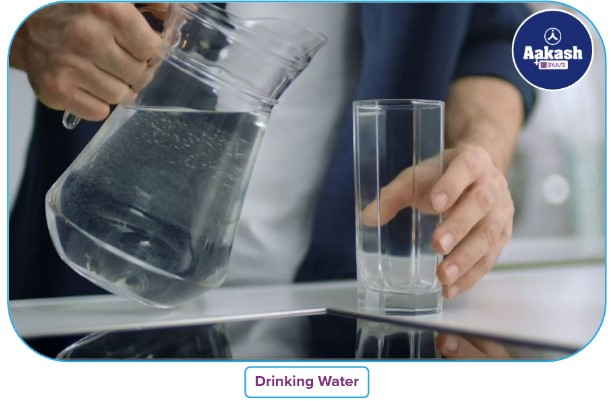Can you suggest any method to detect lead content in water?

Well, you certainly can after going through the concept page. One of the most basic methodologies to check for the amount of impurity in a solution is Gravimetry analysis.

Imagine you went to a laboratory and there was a 100 ml unknown solution. Now you want to know how much impurity is present in the above solution.

How will you proceed to find out the amount of impurity? Which method is best suited for this experiment?

Table of Content:

• Gravimetric Analysis:
• Principle of Gravimetric Analysis:
• Steps to Perform Gravimetric Analysis:
• Example of Gravimetric Analysis:
• Types of Gravimetric Analysis:
• Limitations of Gravimetric method:
• Difference between Gravimetric and Volumetric analysis:
• Practice Problems:

## Gravimetric Analysis:

Gravimetric analysis is one of the commonly used quantitative methods in analytical chemistry, the other being the volumetric method. In gravimetric analysis, the amount of an ion present in an analyte is estimated based on the parameter of mass.

## Principle of Gravimetric Analysis:

The principle of gravimetric analysis is based on the estimation of the mass percent of an ion in an impure compound of known quantity by determining the mass of the same ion in a pure compound. In order to determine the mass, the ion of interest needs to be completely isolated. This isolation of ions is done with the help of precipitation. Typical procedures used in gravimetric analysis include:

• Making a solution with a known amount of the sample analyte.
• Separation of the desired ion/element/radical in pure forms by various separation methods.
• After the ion has been separated, weigh the amount of the pure insoluble compound formed.
• Calculating the value of the individual component of interest, based on the weight of the compound observed.

## Steps to Perform Gravimetric Analysis:

• Insert the sample analyte into a weighing bottle. The sample in the weighing bottle should be left without a lid to dry in an oven and then be cooled in a desiccator..
• Take a known amount of the sample and dissolve it in water after the sample has been fully dried and cooled.
• Precipitation is the technique that is used the most frequently to separate the ion from the sample out of all the methods that are available. Add a precipitating agent to the sample's solution so that it will create an insoluble complex with the target ion while leaving the other components in a soluble state.
• The solution can alternatively be heated to enlarge the insoluble complex for simpler filtration. Digestion is the term for this action.
• Add a few drops of the precipitating agent to see if the insoluble complex is still forming at the tip of the drop to see if the digestion has finished. This step is necessary to make sure that all of the ions of interest in the sample have precipitated and nothing has been left behind.
• Filter the solution containing the insoluble precipitate using a Buchner's funnel while maintaining vacuum.
• Make sure there aren't any traces of the solution left on the beaker's walls by giving it a couple of thorough washes.
• Keep the filter paper for drying once all of the precipitate has been collected on it.
• To get precise results, be sure to completely dry the product.
• Once the product has dried, weigh it to calculate the mass of the formed ion using the stoichiometry of the reaction.
• This calculated mass value can be used to calculate the mass of the same ion in the unidentified sample.

## Example of Gravimetric Analysis:

In order to determine the amount of barium present in the known sample of barium chloride, barium chloride solution of unknown volume can be treated with sulfuric acid to form an insoluble complex of barium sulfate. This barium sulfate can later be used to estimate the amount of barium in the precipitate and subsequently determine the barium's mass percentage in barium chloride.

It is important to note while choosing a precipitating agent; it should be such that it forms a pure insoluble complex with the component of interest and can be easily filtered.

## Types of Gravimetric Analysis:

There are four types of gravimetric analysis based on the method of separation employed in the process. They are:

1. Precipitation Method:

An analytical method called precipitation gravimetry uses a precipitation reaction to separate ions from a solution. Precipitant or precipitating agent refers to the substance that is added to produce precipitation. Filtration can be used to separate the solid precipitate from the liquid components, after which the mass of the solid and the balanced chemical equation can be used to determine the quantity or concentration of ionic compounds in the solution.

2. Volatilization Method:

In volatilization gravimetry, the sample is heated or chemically broken down to separate the components of our mixture. Any volatile compounds are separated out by the heating or chemical decomposition, which causes a change in mass that can be measured.

3. Electrogravimetry Method:

The principle of electrogravimetry is similar to electroplating; here, the metal ions are isolated from the solution by depositing on the surface of the electrode.

4. Thermogravimetric Method:

In this the mass of the sample is determined based on the observed changes in chemical and physical properties over a period of time of varying temperature.

If the procedures are carefully followed, it provides incredibly accurate analysis. With six-figure accuracy, it is used to calculate the atomic masses of numerous elements. It doesn't call for a set of standards to calculate an unknown and leaves little room for instrumental error.

## Limitations of Gravimetric method:

It typically only allows for the analysis of one element, or a small number of elements, at once. contrasting modern gas chromatography and dynamic flash combustion with conventional combustion analysis.

## Practice Problems:

Q.1 Which analytical technique is based on the precipitate weight?

a. Gravimetry
b. Precipitation titration
c. Acid base titration
d. Conductometric titration

Gravimetric analysis is one of the commonly used quantitative methods in analytical chemistry, the other being the volumetric method. In gravimetric analysis, the amount of an ion present in an analyte is estimated based on the parameter of mass.

Q.2 Which of the following is similar to electroplating?

a. Volatilization
b. Precipitation
c. Electrogravimetric
d. Thermogravimetric

The principle of electrogravimetry is similar to electroplating; here, the metal ions are isolated from the solution by depositing on the surface of the electrode.

Q.3 Which of the following units would not be appropriate for volumetric analysis?

a.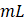b.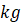c.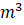d.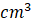The final result of a volumetric analysis is presented in milliliters (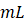), occasionally in microliters (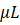), and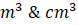can also be used to represent volume.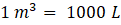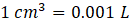Q.4 How many types of gravimetric analysis are there?

a. 2
b. 3
c. 5
d. 4

There are four types of gravimetric analysis based on the method of separation employed in the process. They are:

1. Precipitation method.
2. Volatilization method.
3. Electrogravimetry method.
4. Thermogravimetric method.

Q1. What aspects determine a gravimetric analysis by precipitation's success?
Answer: Two crucial characteristics are present in all precipitation gravimetric analysis. In order for the precipitate mass to accurately reflect that of the analyte, it must first have a low solubility, high purity, and known composition. Second, the precipitate and reaction mixture must be simple to separate.

Q2. In gravimetric analysis, why is HCl added?
Answer: The addition of HCl prevents the formation of insoluble barium salts from other anions, such as phosphate, fluoride, or carbonate, which the solution may contain.

Q3. In gravimetric analysis, what kind of filter paper is employed?
Answer: For quantitative and gravimetric analysis, ash-free filter paper, also known as quantitative filter paper, is employed. In order to achieve high purity and make the paper ash-free during production, manufacturers use acid.

Q4. Why can't we perform this gravimetric analysis using tap water?
Answer: Because impure water contains other ions that could interfere with the formation of a chloride precipitate or form a precipitate with chlorides, making calculations of the mass of the chlorine and gravimetric analysis inaccurate, distilled water must be used when analyzing chlorides.

Related Topics:Talk to our expert
Resend OTP Timer =
By submitting up, I agree to receive all the Whatsapp communication on my registered number and Aakash terms and conditions and privacy policy